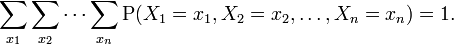### Introduction

The joint probability distribution for X and Y defines the probability of events defined in terms of both X and Y. As defined in the form below:where by the above represents the probability that event x and y occur at the same time.

The cumulative distribution function for a joint probability distribution is given by:In the case of only two random variables, this is called a bivariate distribution, but the concept generalises to any number of random variables, giving a multivariate distribution. The equation for joint probability is different for both dependent and independent events.

### Discrete Case

The joint probability function of two discrete random variables is equal to (Similar to Bayes' theorem):In general, the joint probability distribution of n discrete random variables X1 , X2 , ... ,Xn is equal to:This identity is known as the chain rule of probability.

Since these are probabilities, we have:generalising for n discrete random variables X1 , X2 , ... ,Xn :### Continuous Case

Similarly for continuous random variables, the joint probability density function can be written as fX,Y(x, y) and this is :where fY|X(y|x) and fX|Y(x|y) give the conditional distributions of Y given X=x and of X given Y=y respectively, and fX(x) and fY(y) give the marginal distributions for X and Y respectively.

Again, since these are probability distributions, one has:### Mixed Case

In some situations X is continuous but Y is discrete. For example, in a logistic regression, one may wish to predict the probability of a binary outcome Y conditional on the value of a continuously distributed X. In this case, (X, Y) has neither a probability density function nor a probability mass function in the sense of the terms given above. On the other hand, a "mixed joint density" can be defined in either of two ways:Formally, fX,Y(x , y) is the probability density function of (X, Y) with respect to the product measure on the respective supports of X and Y. Either of these two decompositions can then be used to recover the joint cumulative distribution function:The definition generalises to a mixture of arbitrary numbers of discrete and continuous random variables.

• N/A